Related Articles
Excel VBA | sum() functions
• Last Updated : 20 Jun, 2018

Visual Basic for Applications (VBA) is the programming language of Excel and other offices. It is an event-driven programming language from Microsoft.

With Excel VBA one can automate many tasks in excel and all other office software. It helps in generating reports, preparing various charts, graphs and moreover, it performs calculation using its various functions. Let’s see Sum functions in Excel.

1. SUM: Adds all the numbers in a range of cells.

Syntax:

```=SUM(number1, number2…)
```

Here,
number 1: This is the first number in your cell to add. You can specify it upto 255 numbers.
number 2: This is an Optional field. You can add upto 255 numbers.

Example: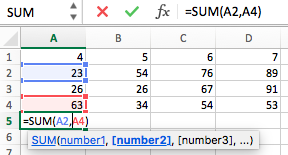Output: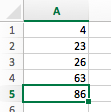2. SUMIF: Allows to add the cells depending upon the criteria you’ve provided.

Syntax:

```=SUMIF(range, criteria, sum_range)
```

range: It is the range of cells which you want to evaluate.
The cells in range can be numbers, names or arrays or any other reference that contains numbers.

criteria: It should be in the form of a number or any expression which defines which all cells to be added.

```For instance, “mango”, C6, “<35”
```

sum_range: This field is Optional. If this is not mentioned, Excel will add only those cells on which criteria is applied, which are specified in the range argument.

Example: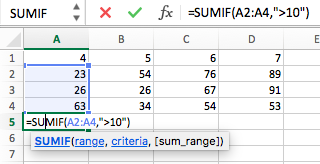Output: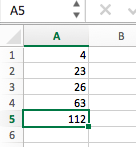3. SUMIFS: Allows you to add all the arguments that meet multiple criteria.

Syntax:

```=SUMIFS(sum_range, criteria_range1, criteria1 [criteria_range2, criteria2], …)
```

sum_range: (Required) Actual cells to sum.

criteria_range1: (Required) criteria_range1 and criteria1 makes up a search pair through which a range is searched for some specific criteria. When elements in the range are located, their corresponding values in sum_range are added.

criteria1: This defines which all cells in criteria_range1 are to be added.

```For instance, criteria can be like this, “>32”, “apple”, “D7”
```

Example: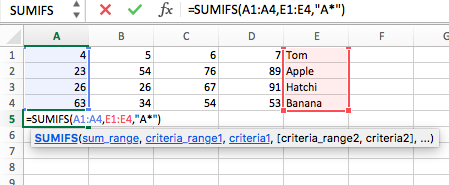Output: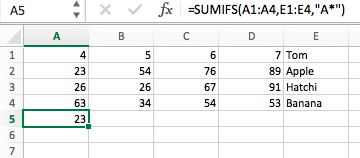My Personal Notes arrow_drop_up
Recommended Articles
Page :